scale_x_continuous() and scale_y_continuous() are the default scales for continuous x and y aesthetics. There are three variants that set the trans argument for commonly used transformations: scale_*_log10(), scale_*_sqrt() and scale_*_reverse().

## Usage

scale_x_continuous(
name = waiver(),
breaks = waiver(),
minor_breaks = waiver(),
n.breaks = NULL,
labels = waiver(),
limits = NULL,
expand = waiver(),
oob = censor,
na.value = NA_real_,
trans = "identity",
guide = waiver(),
position = "bottom",
sec.axis = waiver()
)

scale_y_continuous(
name = waiver(),
breaks = waiver(),
minor_breaks = waiver(),
n.breaks = NULL,
labels = waiver(),
limits = NULL,
expand = waiver(),
oob = censor,
na.value = NA_real_,
trans = "identity",
guide = waiver(),
position = "left",
sec.axis = waiver()
)

scale_x_log10(...)

scale_y_log10(...)

scale_x_reverse(...)

scale_y_reverse(...)

scale_x_sqrt(...)

scale_y_sqrt(...)

## Arguments

name

The name of the scale. Used as the axis or legend title. If waiver(), the default, the name of the scale is taken from the first mapping used for that aesthetic. If NULL, the legend title will be omitted.

breaks

One of:

• NULL for no breaks

• waiver() for the default breaks computed by the transformation object

• A numeric vector of positions

• A function that takes the limits as input and returns breaks as output (e.g., a function returned by scales::extended_breaks()). Also accepts rlang lambda function notation.

minor_breaks

One of:

• NULL for no minor breaks

• waiver() for the default breaks (one minor break between each major break)

• A numeric vector of positions

• A function that given the limits returns a vector of minor breaks. Also accepts rlang lambda function notation.

n.breaks

An integer guiding the number of major breaks. The algorithm may choose a slightly different number to ensure nice break labels. Will only have an effect if breaks = waiver(). Use NULL to use the default number of breaks given by the transformation.

labels

One of:

• NULL for no labels

• waiver() for the default labels computed by the transformation object

• A character vector giving labels (must be same length as breaks)

• An expression vector (must be the same length as breaks). See ?plotmath for details.

• A function that takes the breaks as input and returns labels as output. Also accepts rlang lambda function notation.

limits

One of:

• NULL to use the default scale range

• A numeric vector of length two providing limits of the scale. Use NA to refer to the existing minimum or maximum

• A function that accepts the existing (automatic) limits and returns new limits. Also accepts rlang lambda function notation. Note that setting limits on positional scales will remove data outside of the limits. If the purpose is to zoom, use the limit argument in the coordinate system (see coord_cartesian()).

expand

For position scales, a vector of range expansion constants used to add some padding around the data to ensure that they are placed some distance away from the axes. Use the convenience function expansion() to generate the values for the expand argument. The defaults are to expand the scale by 5% on each side for continuous variables, and by 0.6 units on each side for discrete variables.

oob

One of:

• Function that handles limits outside of the scale limits (out of bounds). Also accepts rlang lambda function notation.

• The default (scales::censor()) replaces out of bounds values with NA.

• scales::squish() for squishing out of bounds values into range.

• scales::squish_infinite() for squishing infinite values into range.

na.value

Missing values will be replaced with this value.

trans

For continuous scales, the name of a transformation object or the object itself. Built-in transformations include "asn", "atanh", "boxcox", "date", "exp", "hms", "identity", "log", "log10", "log1p", "log2", "logit", "modulus", "probability", "probit", "pseudo_log", "reciprocal", "reverse", "sqrt" and "time".

A transformation object bundles together a transform, its inverse, and methods for generating breaks and labels. Transformation objects are defined in the scales package, and are called <name>_trans (e.g., scales::boxcox_trans()). You can create your own transformation with scales::trans_new().

guide

A function used to create a guide or its name. See guides() for more information.

position

For position scales, The position of the axis. left or right for y axes, top or bottom for x axes.

sec.axis

sec_axis() is used to specify a secondary axis.

...

Other arguments passed on to scale_(x|y)_continuous()

## Details

For simple manipulation of labels and limits, you may wish to use labs() and lims() instead.

Other position scales: scale_x_binned(), scale_x_date(), scale_x_discrete()

## Examples

p1 <- ggplot(mpg, aes(displ, hwy)) +
geom_point()
p1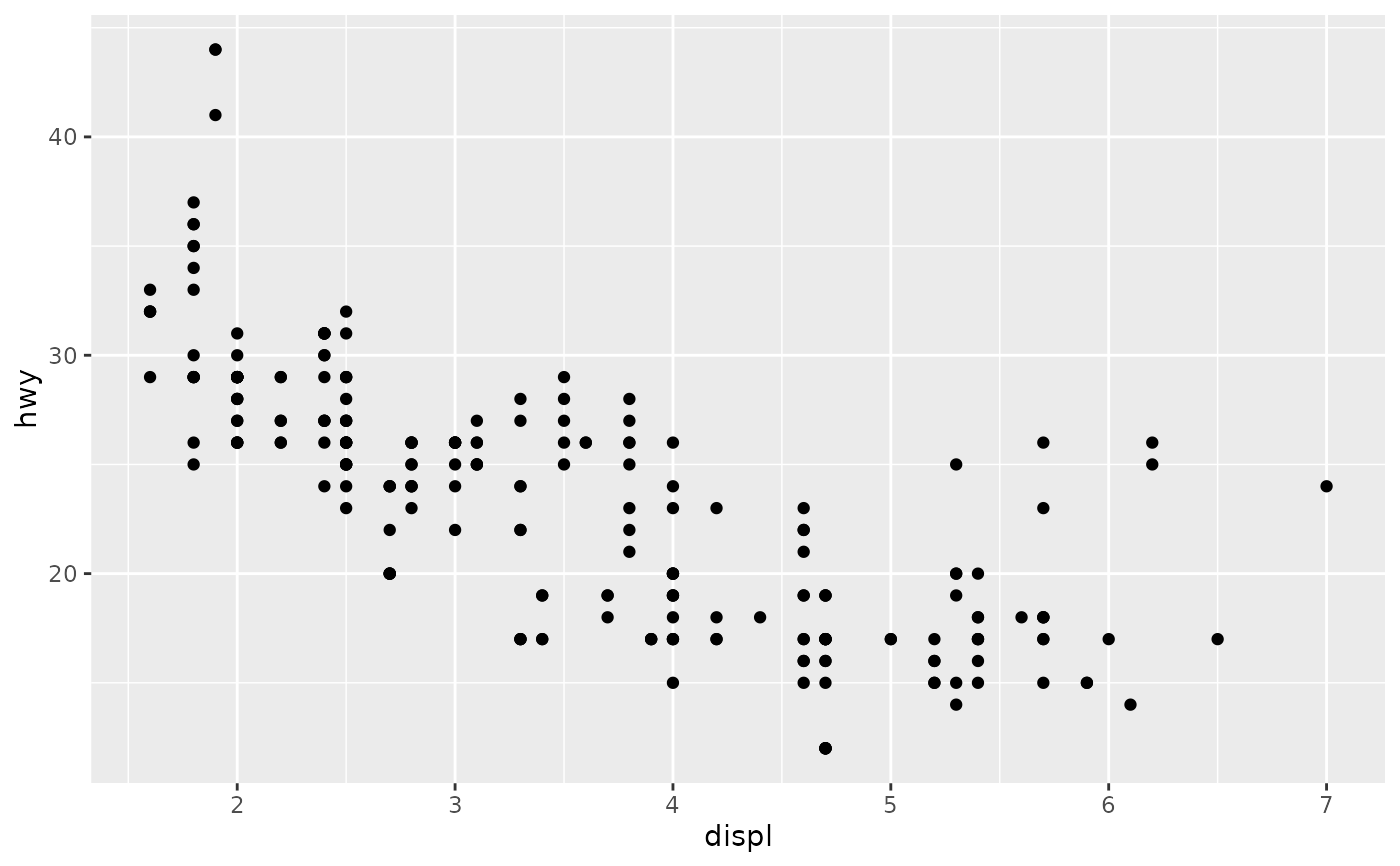# Manipulating the default position scales lets you:
#  * change the axis labels
p1 +
scale_x_continuous("Engine displacement (L)") +
scale_y_continuous("Highway MPG")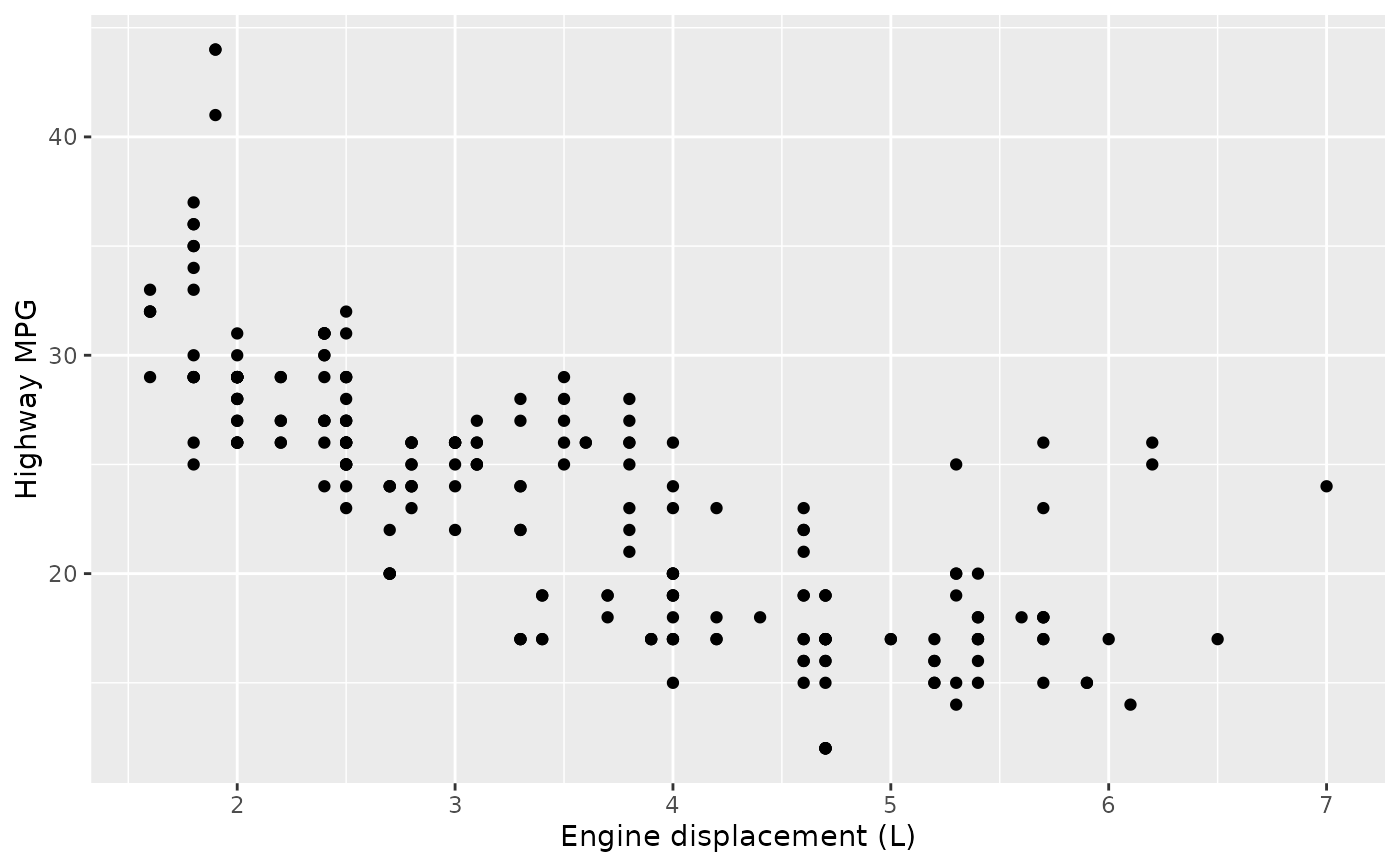# You can also use the short-cut labs().
# Use NULL to suppress axis labels
p1 + labs(x = NULL, y = NULL)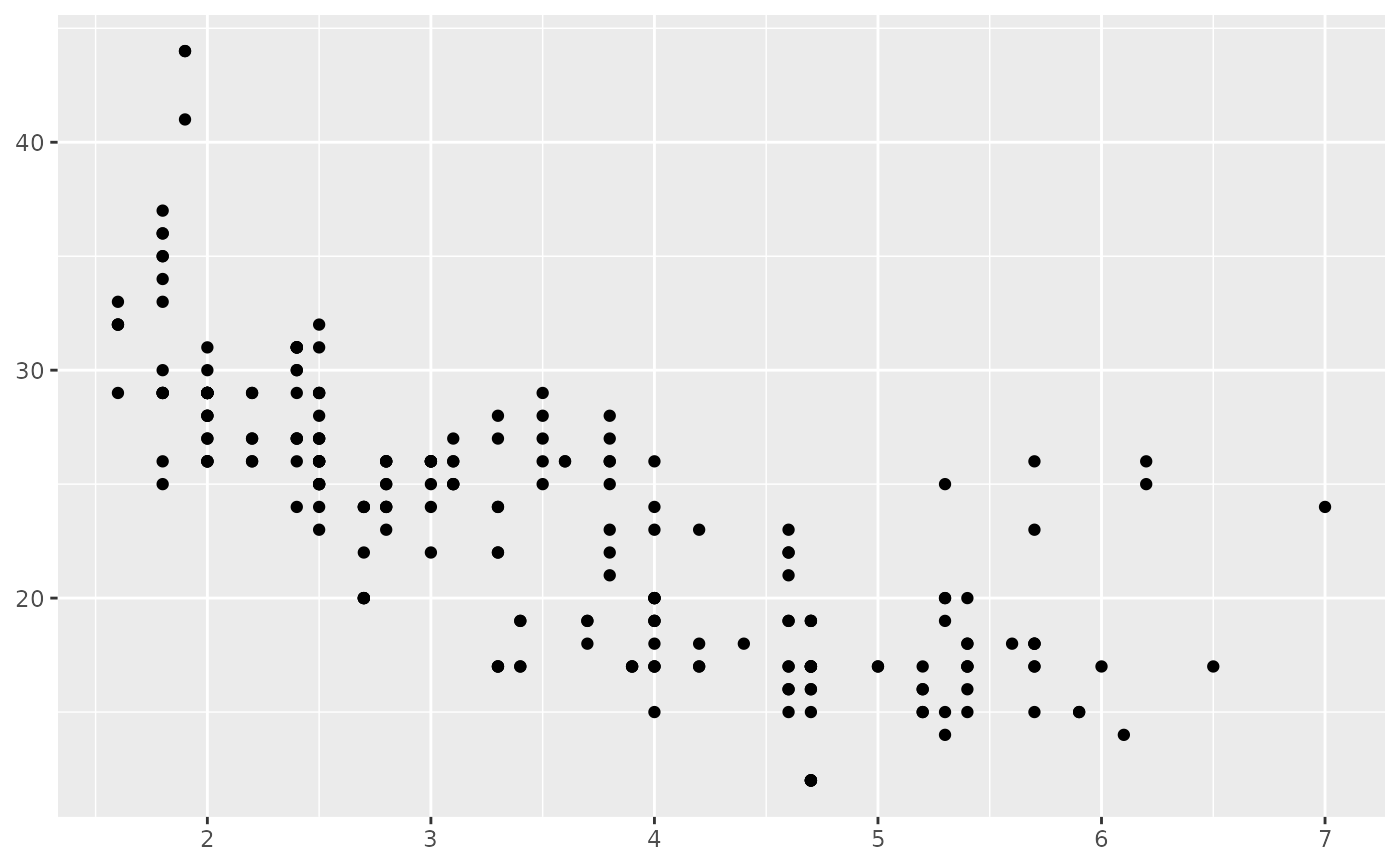#  * modify the axis limits
p1 + scale_x_continuous(limits = c(2, 6))
#> Warning: Removed 27 rows containing missing values (geom_point()).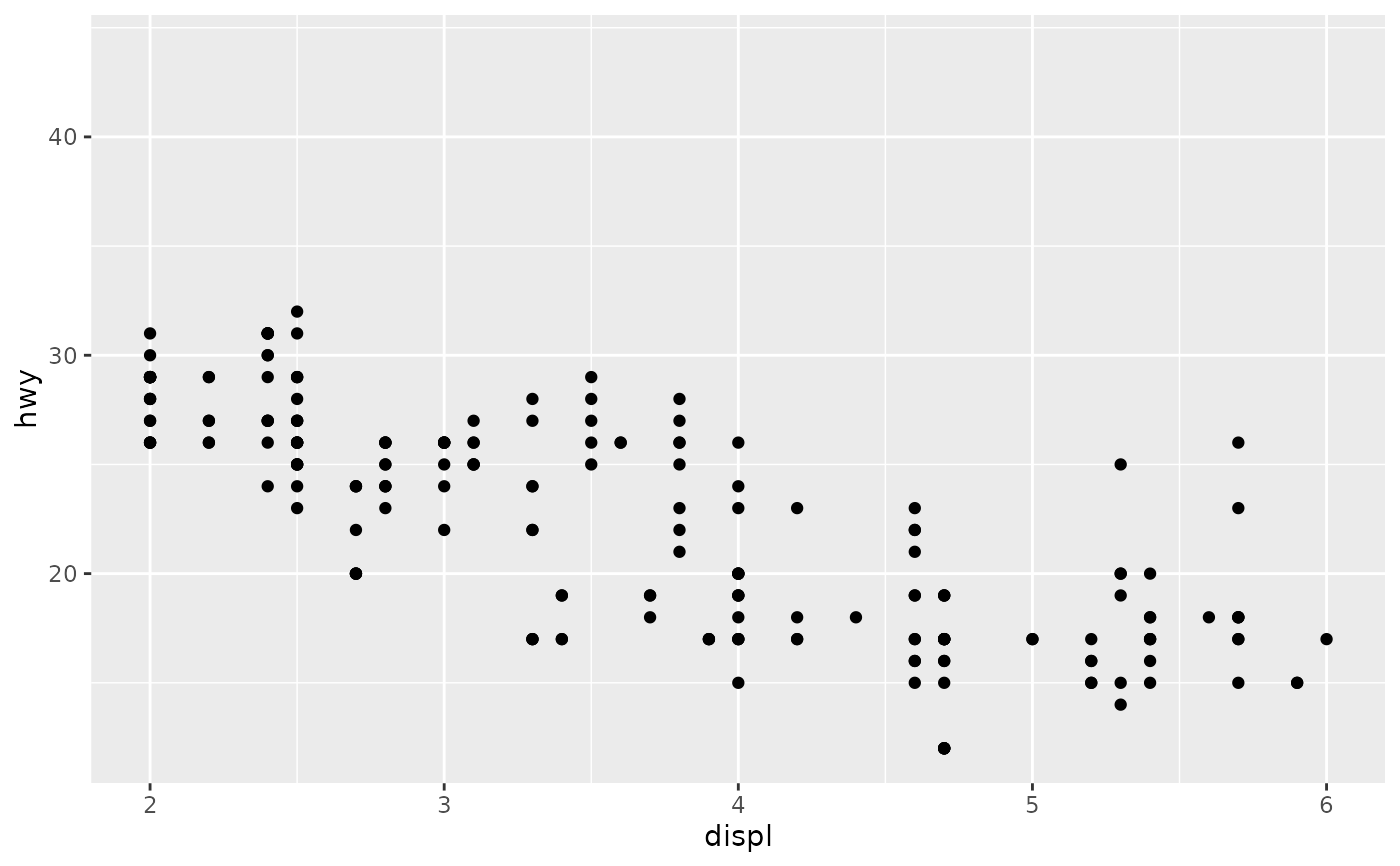p1 + scale_x_continuous(limits = c(0, 10))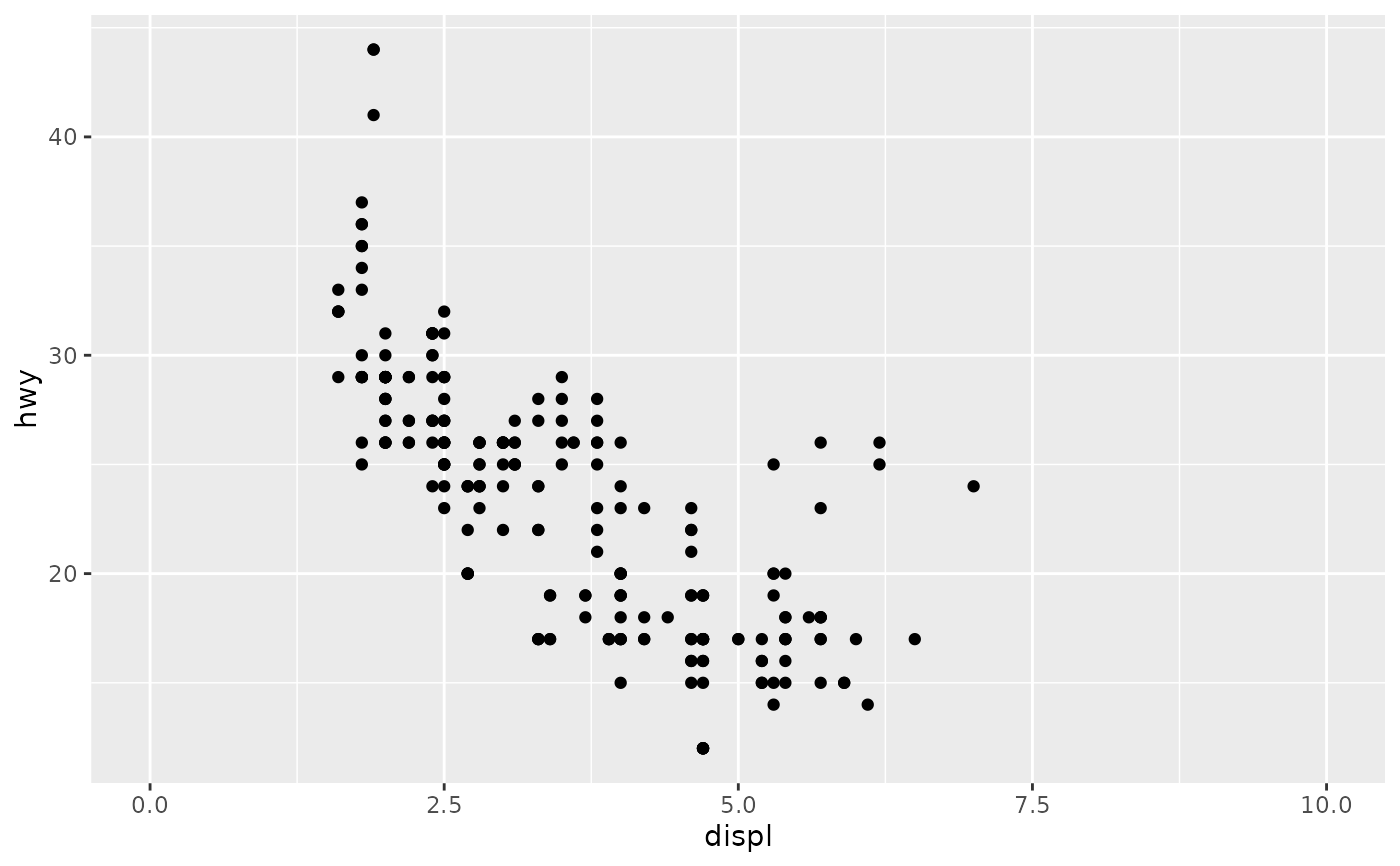# you can also use the short hand functions xlim() and ylim()
p1 + xlim(2, 6)
#> Warning: Removed 27 rows containing missing values (geom_point()).#  * choose where the ticks appear
p1 + scale_x_continuous(breaks = c(2, 4, 6))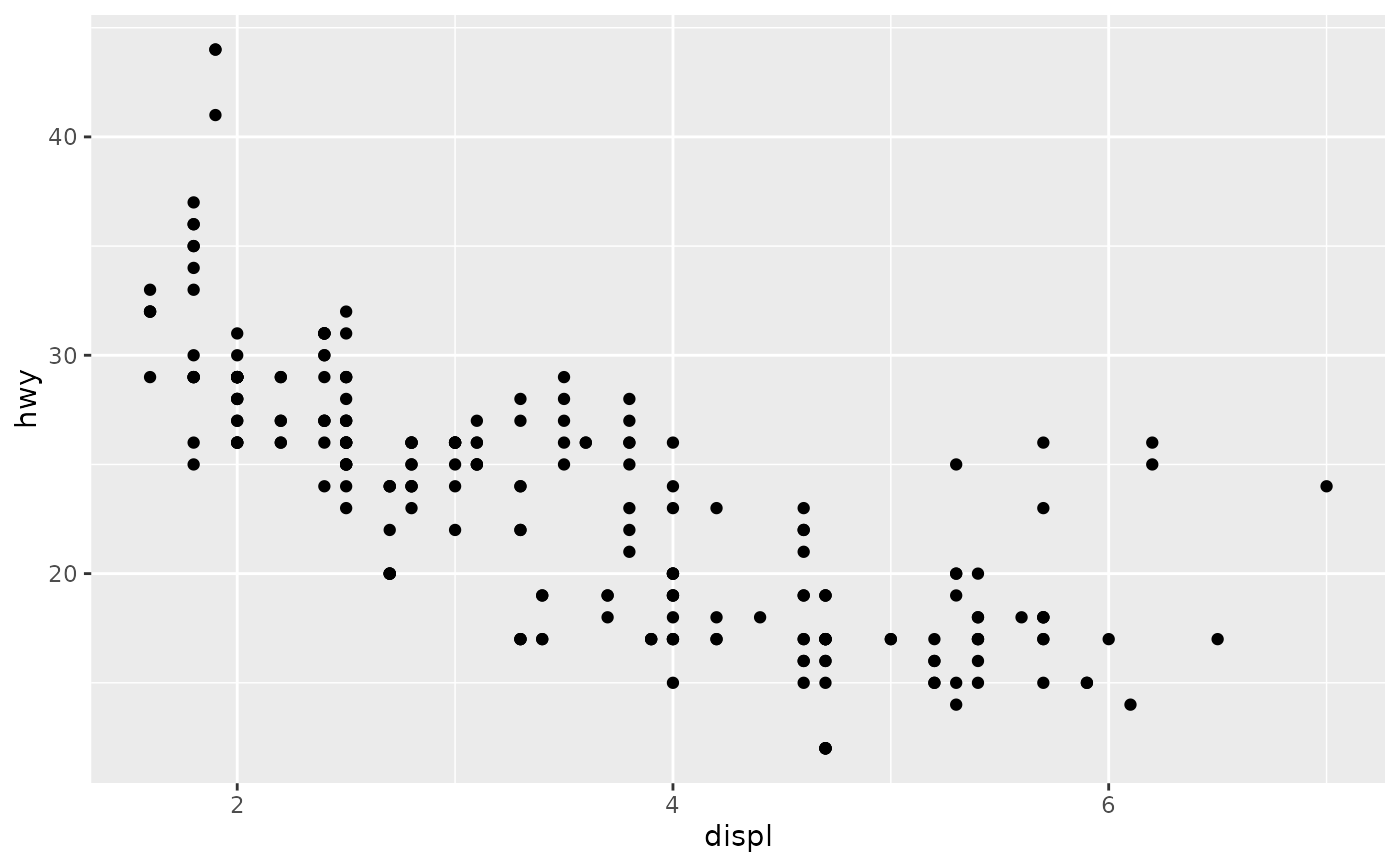#  * choose your own labels
p1 + scale_x_continuous(
breaks = c(2, 4, 6),
label = c("two", "four", "six")
)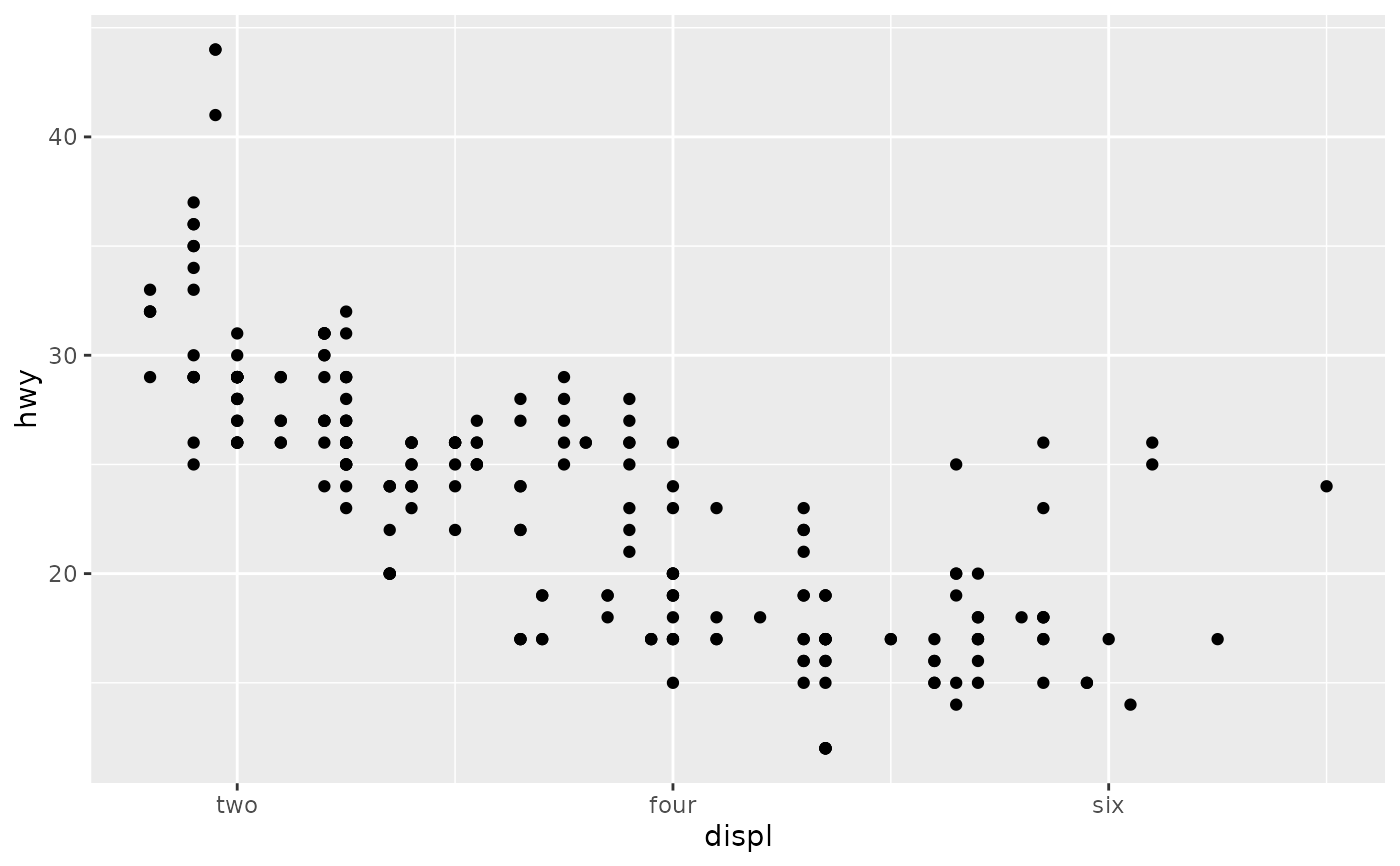# Typically you'll pass a function to the labels argument.
# Some common formats are built into the scales package:
set.seed(1)
df <- data.frame(
x = rnorm(10) * 100000,
y = seq(0, 1, length.out = 10)
)
p2 <- ggplot(df, aes(x, y)) + geom_point()
p2 + scale_y_continuous(labels = scales::percent)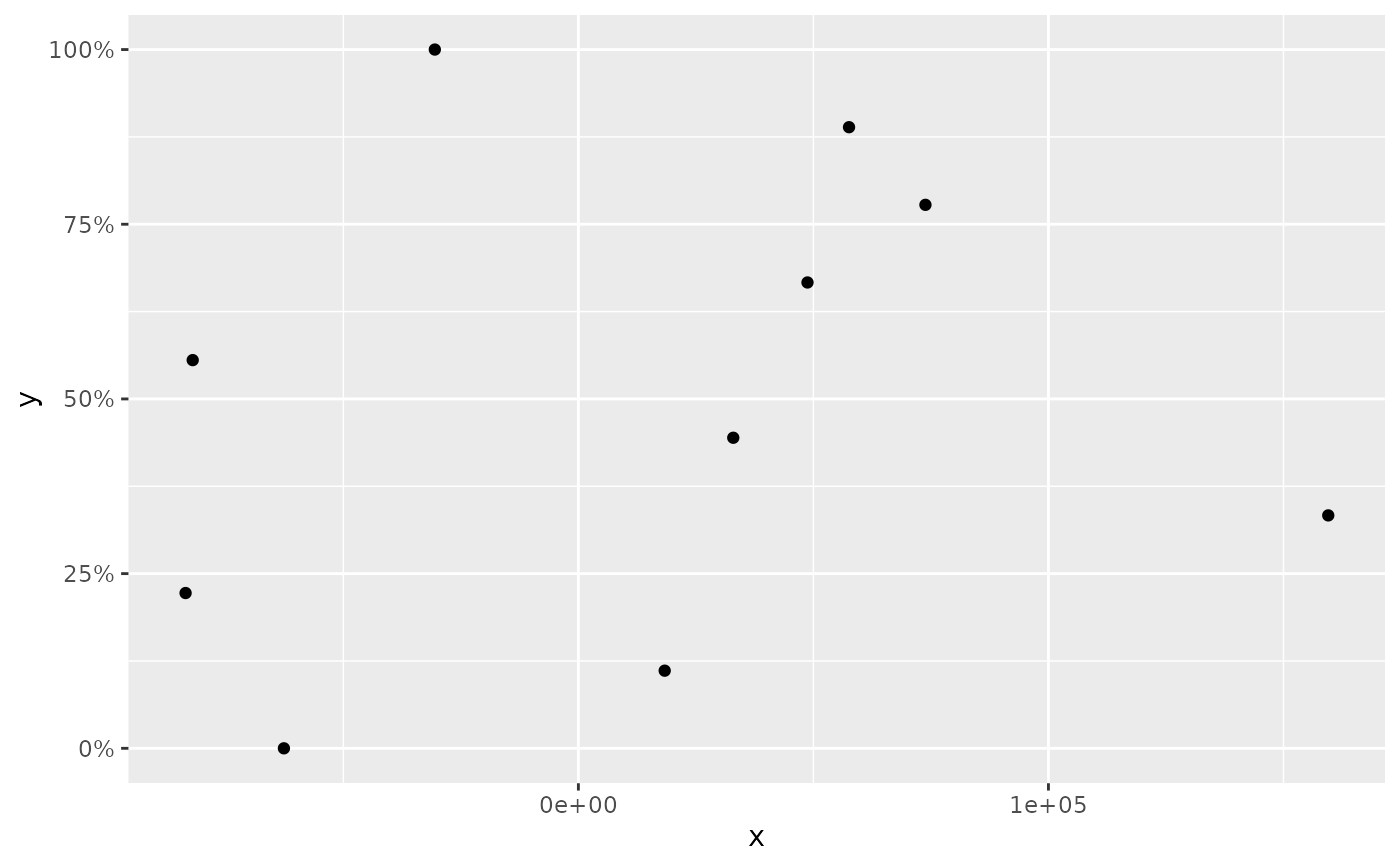p2 + scale_y_continuous(labels = scales::dollar)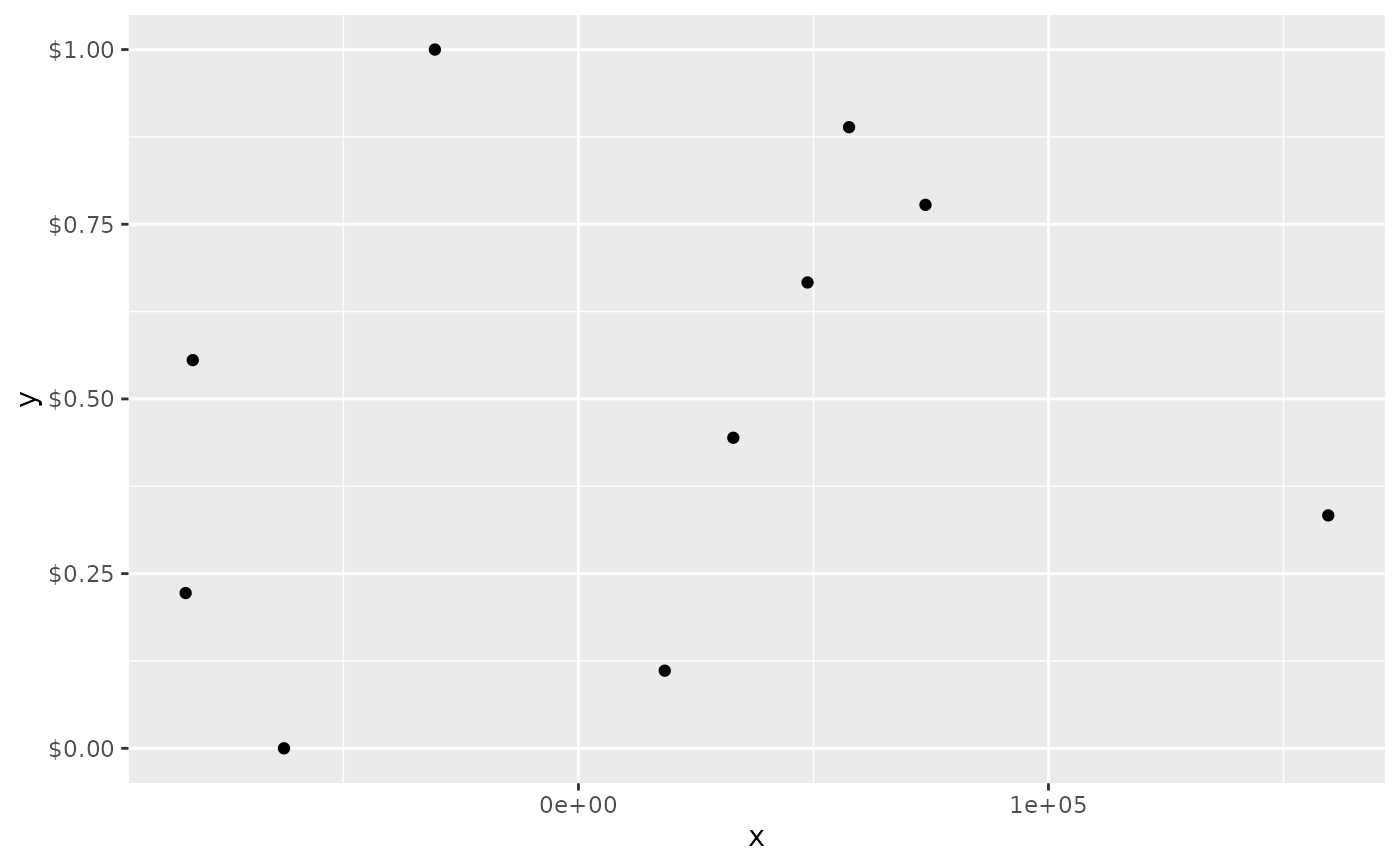p2 + scale_x_continuous(labels = scales::comma)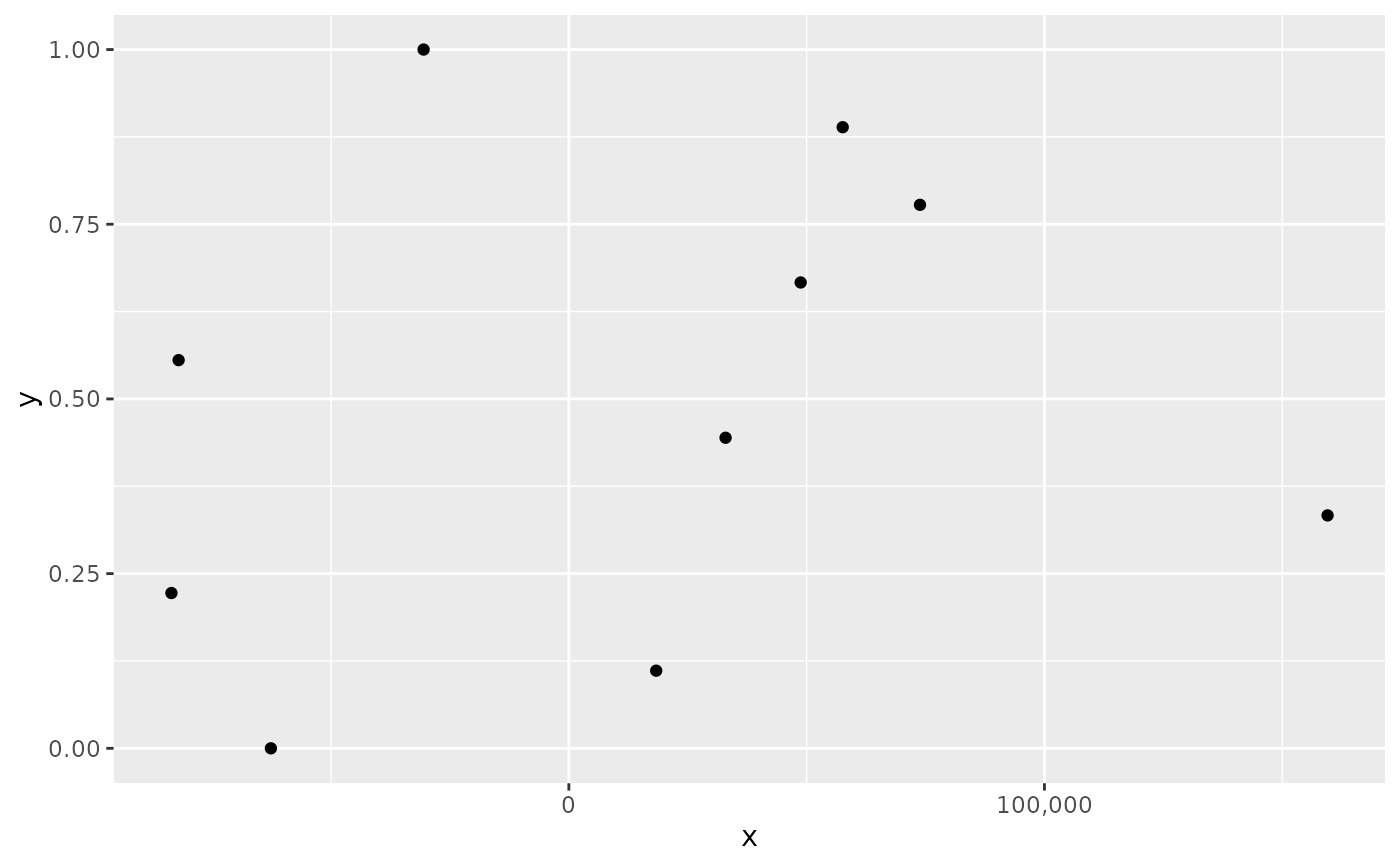# You can also override the default linear mapping by using a
# transformation. There are three shortcuts:
p1 + scale_y_log10()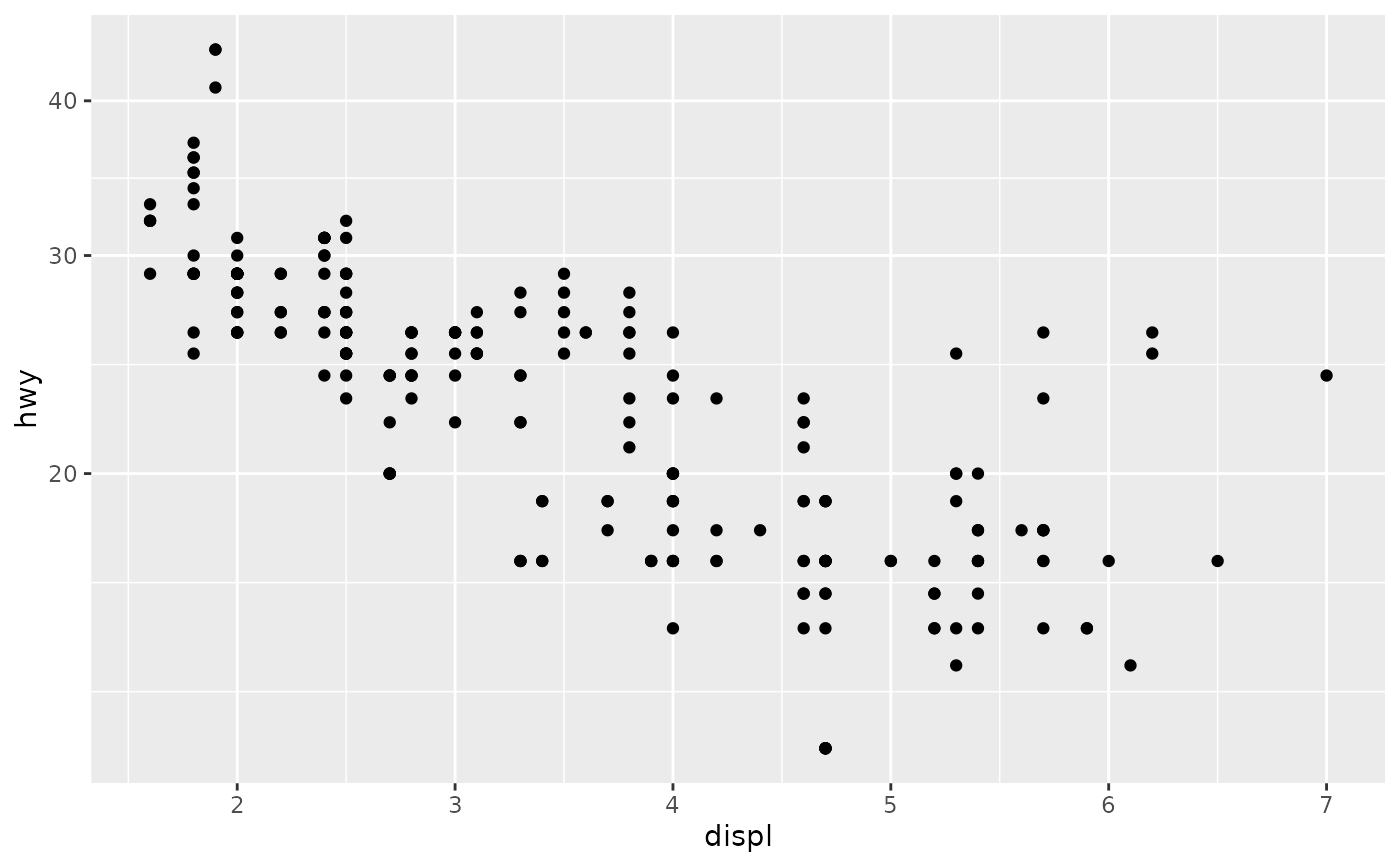p1 + scale_y_sqrt()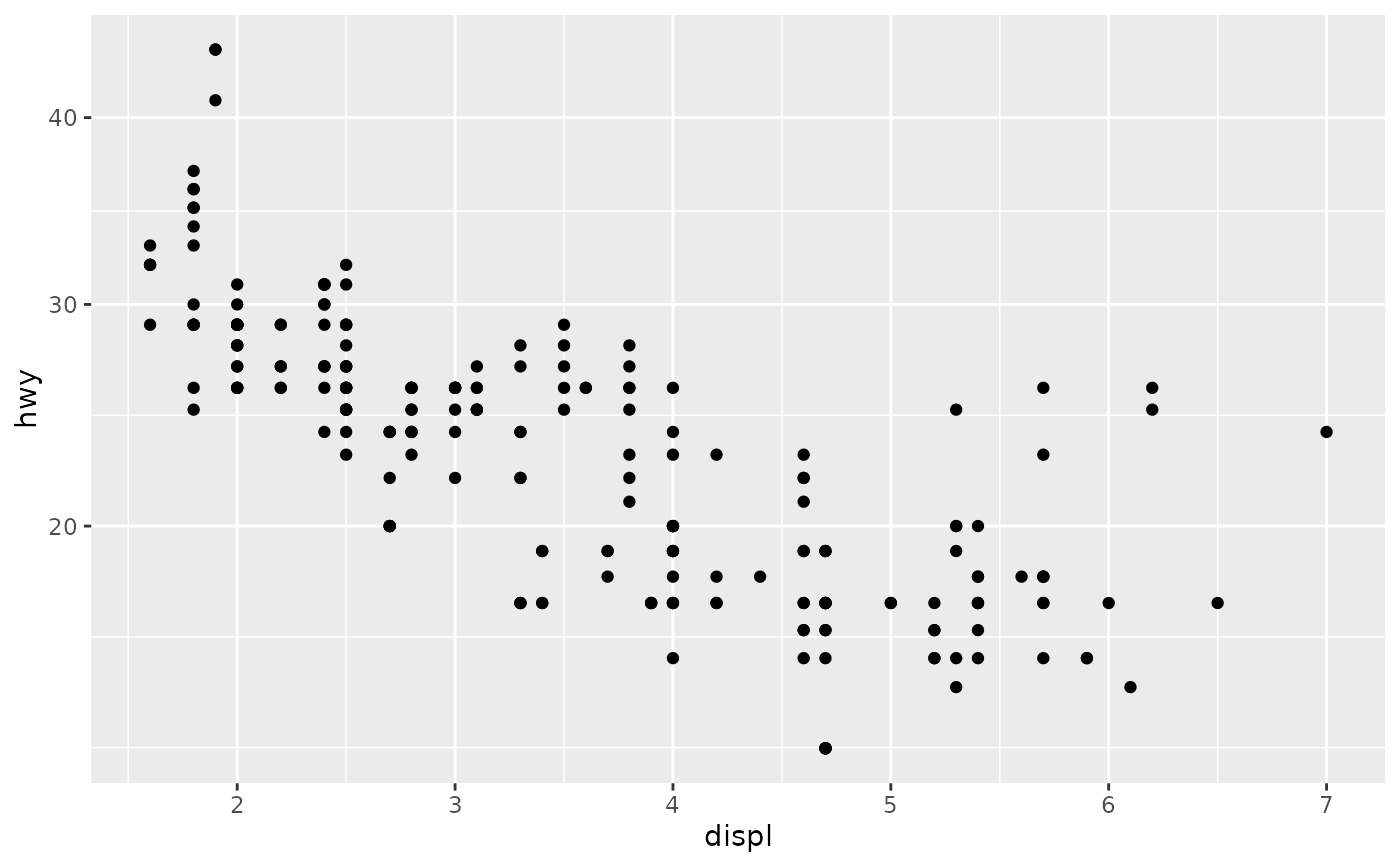p1 + scale_y_reverse()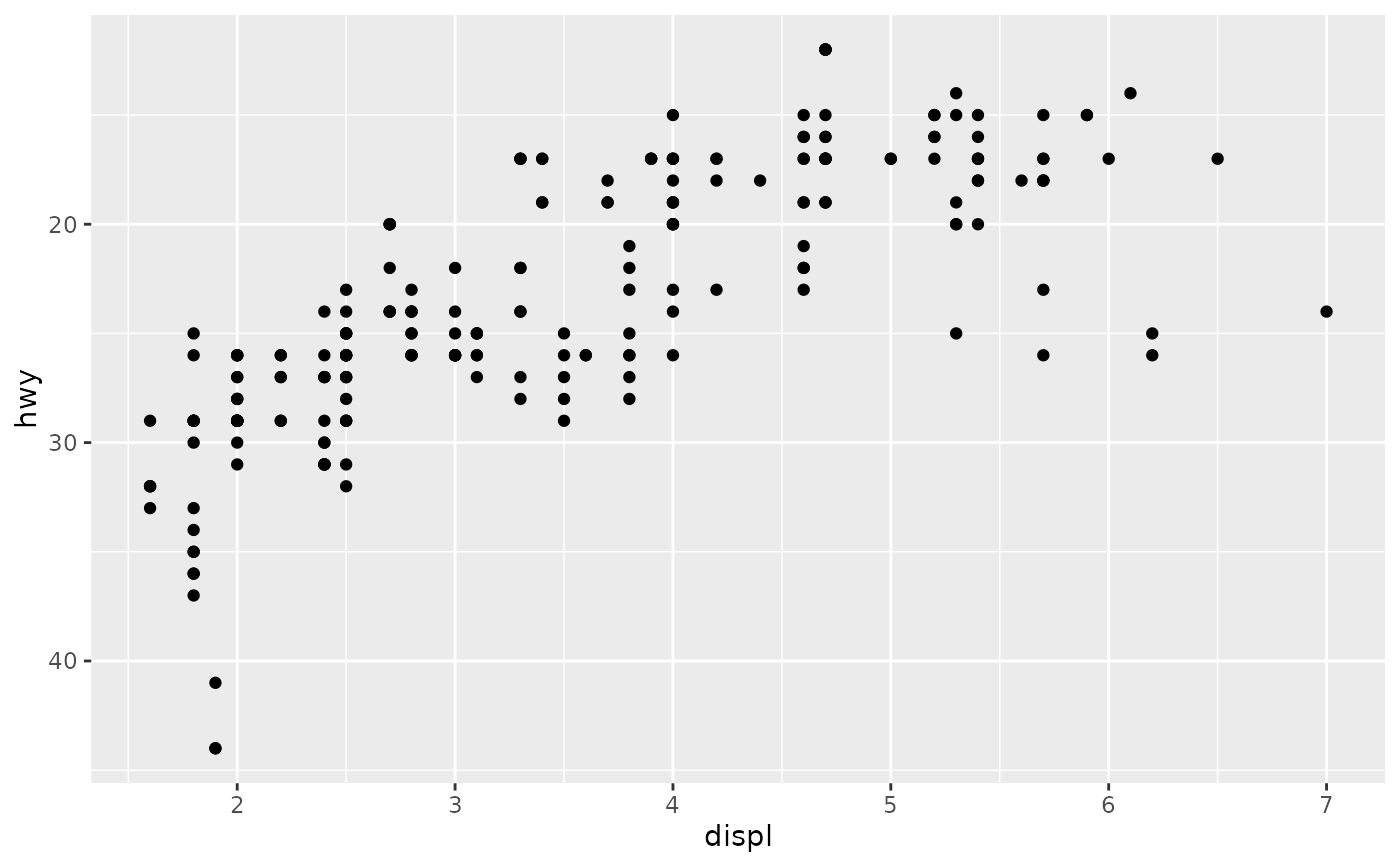# Or you can supply a transformation in the trans argument:
p1 + scale_y_continuous(trans = scales::reciprocal_trans())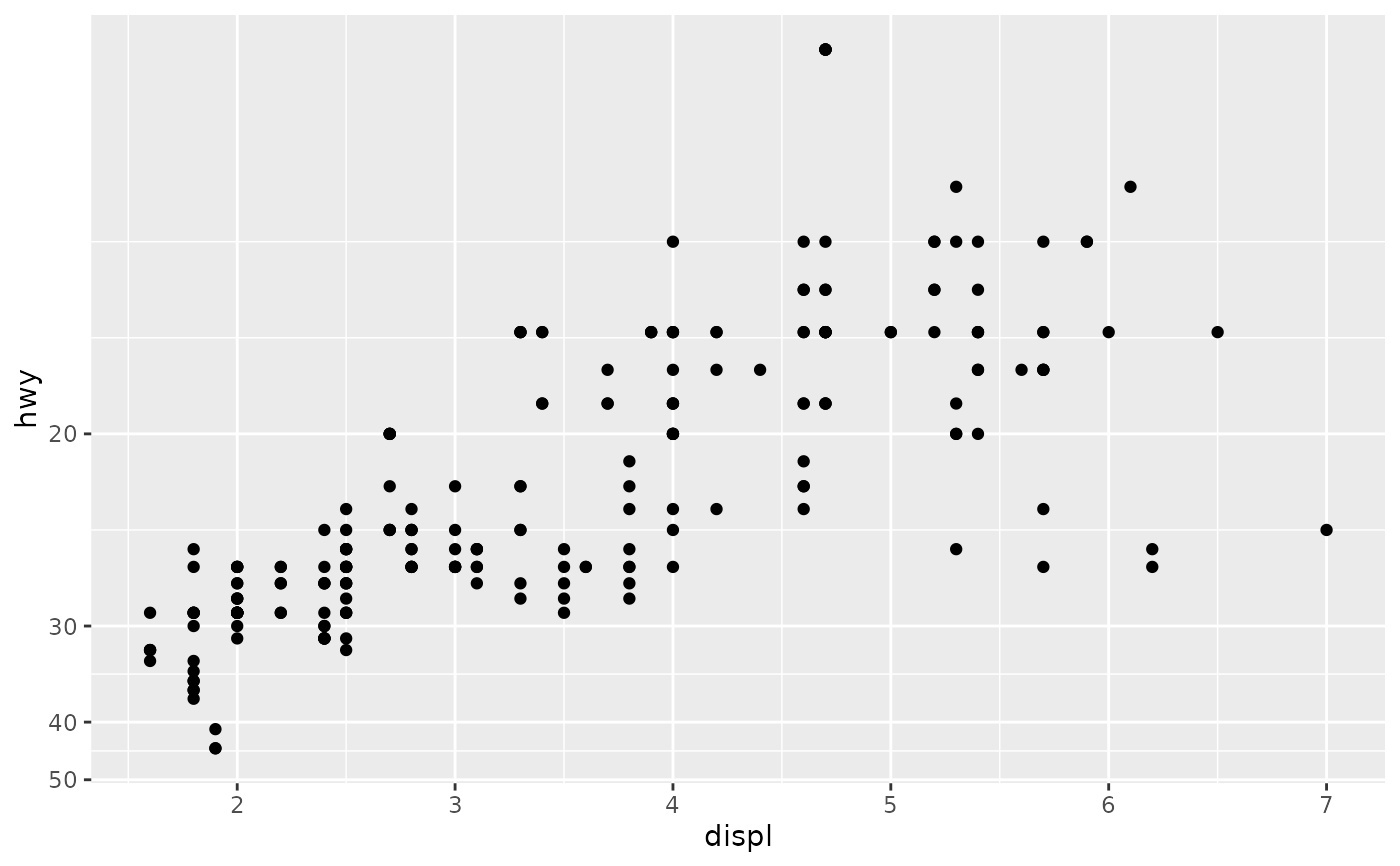# You can also create your own. See ?scales::trans_new